TitleIntermediate Algebra Tutorial 4: Operations on Numbers

WTAMU > Virtual Math Lab > Intermediate AlgebraLearning Objectives

 After completing this tutorial, you should be able to: Add and subtract real numbers. Multiply and divide real numbers. Evaluate a numeric exponential expression. Evaluate a numeric radical expression. Use the order of operations appropriately.Introduction

 This tutorial reviews the adding, subtracting, multiplying, and dividing of real numbers as well as using exponents and roots. Once we have that all down, we will put it all together using the order of operations.   I have the utmost confidence that you are familiar with these operations, but sometimes the rules for negative numbers (yuck!) get a little mixed up from time to time.  So, it is good to go over them to make sure you have them down.  This is a very good topic to have down, because real numbers are EVERYWHERE.  Even in this day and age of calculators, it is very important to know these basic rules of operations on real numbers.  Even if you are using a calculator, you are the one that is putting the information into it, so you need to know things like when you are subtracting versus multiplying and the order that you need to put it in.  Also, if you are using a calculator you should have a rough idea as to what the answer should be. You never know, you may hit a wrong key and get a wrong answer (it happens to the best of us).  Also, your batteries in your calculator may run out and you may have to do a problem by hand (scary!!!).   You want to be prepared for those Murphy's Law moments.   We will start with the individual operations and then mix ‘em up using order of operations.Tutorial

 Adding Real Numbers  with the Same Sign

 Step 1:   Add the absolute values.   Step 2:   Attach their common sign to sum .Example 1:   Find the sum -6 + (-8).

 -6 + (-8) = -14  The sum of the absolute values would be 14 and their common sign is -.  That is how we get the answer of -14.  You can also think of this as money - I know we can all relate to that.  Think of the negative as a loss.  In this example, you can think of it as having lost 6 dollars and then having lost another 8 dollars for a total loss of 14 dollars.Example 2:   Find the sum  -5.5 + (-8.7).

 -5.5 + (-8.7) = -14.2 The sum of the absolute values would be 14.2 and their common sign is -. That is how we get the answer of -14.2. You can also think of this as money - I know we can all relate to that.  Think of the negative as a loss.  In this example, you can think of it as having lost 5.5 dollars and then having lost another 8.7 dollars for a total loss of 14.2 dollars.

 Adding Real Numbers  with Opposite Signs

 Step 1:   Take the difference of the absolute values.    Step 2:   Attach the sign of the number that has the higher absolute value.Example 3:   Find the sum  -8 + 6.

 -8 + 6 = -2.  The difference between 8 and 6 is 2 and the sign of 8 (the larger absolute value) is -.  That is how we get the answer of -2.  Thinking in terms of money: we lost 8 dollars and got back 6 dollars, so we are still in the hole 2 dollars.Example 4:   Find the sum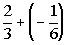.*Mult. top and bottom of first fraction by 2 to get the LCD of 6     *Take the difference of the numerators and write over common denominator 6 *Reduce fraction

 The difference between 4/6 and 1/6 is 3/6 = 1/2 and the sign of 4/6 (the larger absolute value) is +.  That is how we get the answer of 1/2. Thinking in terms of money: we had 2/3 of a dollar and lost 1/6 of a dollar, so we would come out ahead 1/2 of a dollar. Note that if you need help on fractions, click on this link:  Fractions

 Subtracting Real Numbers a - b = a + (-b)    or  a - (-b) = a + b

 In other words, to subtract b, you add the opposite of b.  Now, you do not have to write it out like this if you are already comfortable with it. This just gives you the thought behind it.Example 5: Find the difference   -3 - 5.

 -3 - 5 = -3 + (-5) = -8.  Subtracting 5 is the same as adding a -5.  Once it is written as addition, I just follow the rules for addition, as shown above, to complete for an answer of -8.Example 6: Find the difference  -3 - (-5).

 -3 - (-5) = -3 + 5 = 2.  Subtracting -5 is the same as adding 5.  Once it is written as addition, I just follow the rules for addition, as shown above, to complete for an answer of 2.

 Multiplying or Dividing Real Numbers

Since dividing is the same as multiplying by the reciprocal, dividing and multiplying have the same sign rules.

Step 1:   Multiply or divide their absolute values.

Step 2:   Put the correct sign.

 If the two numbers have the same sign, the product or quotient is positive.  If they have opposite signs, the product or quotient is negative.Example 7:  Find the product  (-4)(3).

 (-4)(3) = -12.  The product of the absolute values 4 x 3 is 12 and they have opposite signs, so our answer is -12.Example 8:  Find the product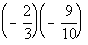.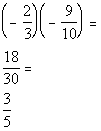*Mult. num. together *Mult. den. together *(-)(-) = (+) *Reduce fraction

 The product of the absolute values -2/3 x -9/10 is 18/30 = 3/5 and they have the same sign, so that is how we get the answer 3/5. Note that if you need help on fractions, click on this link:  FractionsExample 9:  Find the quotient   (-10)/(-2).

 (-10)/(-2) = 5  The quotient of the absolute values 10/2 is 5 and they have the same signs, so our answer is 5.Example 10:  Find the quotient.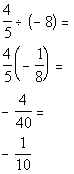*Div. is the same as mult. by reciprocal *Mult. num. together *Mult. den. together *(+)(-) = - *Reduce fraction

 The quotient of the absolute values 4/5 and 8 is 4/40 = 1/10 and they have opposite signs, so our answer is -1/10.   Note that if you need help on fractions, click on this link:  Fractions

 Multiplying by and  Dividing into Zero a(0) = 0 and 0/a = 0   (when a does not equal 0)

 In other words, zero (0) times any real number is zero (0) and zero (0) divided by any real number other than zero (0) is zero (0).Example 11:   Find the product   0(½).

 0(½) = 0  Multiplying any expression by 0 results in an answer of 0.Example 12:   Find the quotient 0/5.

 0/5 = 0  Dividing 0 by any expression other than 0 results in an answer of 0.

 Dividing by Zero a/0 is undefined

 Zero (0) does not go into any number, so whenever you are dividing by zero (0) your answer is undefined.Example 13:   Find the quotient 5/0.

 5/0 = undefined.  Dividing by 0 results in an undefined answer.

 Definition of Exponents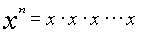(note there are n x's in the product) x = base,    n = exponent

 Exponents are another way to write multiplication.  The exponent tells you how many times a base appears in a PRODUCT.Example 14:   Evaluate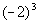.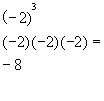*Write the base -2 in a product 3 times *MultiplyExample 15:   Evaluate.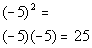*Write the base -5 in a product 2 times *Multiply

 Note how I included the - when I expanded this problem out.  If the - is inside the ( ) of an exponent, then it is included as part of the base.Example 16:   Evaluate.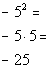*Negate 5 squared *Put a - in front of 5 written in a product 2 times *Multiply

 Hey, this looks a lot like example 15!!!!  It may look alike, but they ARE NOT exactly the same.  Can you see the difference between the two??  Hopefully, you noticed that in example 15, there was a ( ) around the - and the 5.  In this problem, there is no (  ).  This means the - is NOT part of the base, so it will not get expanded like it did in example 15.  It is interpreted as finding the negative or opposite of 5 squared.

 Principal nth Root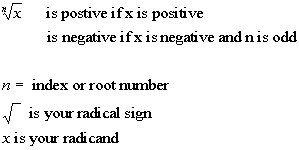Things to note about radicals in general, When there is no index number n, it is understood to be a 2 or square root. When looking for the nth radical or nth root, you want the expression that when you raise it to the nth power you would get the radicand (what is inside the radical sign).Example 17:   Find the root: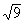.

 The thought behind this is that we are looking for the square root of 9.   This means we are looking for a number that when we square it we get 9.   Since 3 squared is 9, 3 is the square root of 9.  Note that we are only interested in the principal root and since 9 is positive and there is not a sign in front of the radical, our answer is positive 3.  If there had been a negative in front of the radical our answer would have been -3.Example 18:   Find the root: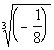.

 Contrary to popular belief, not every radical is a square root.  If there is an index or root number written, make sure you take the appropriate root. Now we are looking for the third or cube root of -1/8, which means we are looking for a number that when we cube it we get -1/8.  Since -1/2 cubed is -1/8, our answer is going to be -1/2.

.

 Order of Operations Please   Parenthesis or grouping symbols Excuse   Exponents (and radicals) My Dear   Multiplication/Division left to right Aunt Sally  Addition/Subtraction left to right

 As you know, life (as well as algebra) is not always as cut and dry like the above examples.  It is very important for you to understand each individual operation first (that is what we were doing in the above examples) and now we will put them together and mix them all about. When you do have more than one mathematical operation, you need to use the order of operations as listed above. You may have already heard of the saying "Please Excuse My Dear Aunt Sally".  It is just a way to help you remember the order you need to go in when applying the order of operations.Example 19:    Simplify.

 Using the Order of Operations we get: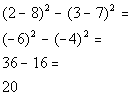*Work inside ( ) *Exponents *SubtractionExample 20:     Simplify.

 An absolute value is like a fancy grouping symbol.  So, if you have one, you need to do what is inside first, before tackling the other operations that are on the outside of it, as shown below.   Using the Order of Operations we get: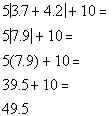*Work inside |   | *Multiply *AddExample 21:   Find the value of the expression when x = -1 and y = 5.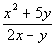When you have a fraction, like the one in this example, there are hidden grouping symbols.  The fraction bar separates the numerator from the denominator.  You need to think of it as if there is a (  ) around the numerator  and one around the denominator.  In other words, when you have a problem like this one, you need to find the numerator and denominator first, before you deal with the fraction bar (division).   Plugging in values for the variables and evaluating the expression using the Order of Operations we get: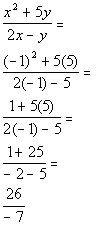*Replace x with -1 and y with 5   *Exponent in num. *Multiply in num. and den. *Add in num. and subtract in den.Practice Problems

 These are practice problems to help bring you to the next level.  It will allow you to check and see if you have an understanding of these types of problems. Math works just like anything else, if you want to get good at it, then you need to practice it.  Even the best athletes and musicians had help along the way and lots of practice, practice, practice, to get good at their sport or instrument.  In fact there is no such thing as too much practice. To get the most out of these, you should work the problem out on your own and then check your answer by clicking on the link for the answer/discussion for that  problem.  At the link you will find the answer as well as any steps that went into finding that answer.Practice Problems 1a - 1c: Find the sum or difference.

 1a. -5 + (-16) (answer/discussion to 1a) 1b.    7 + (-3) (answer/discussion to 1b)

 1c.   -10 - (-2) (answer/discussion to 1c)Practice Problems 2a - 2b: Find the product or quotient.

 2a.   (-4)(9) (answer/discussion to 2a) 2b.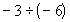(answer/discussion to 2b)Practice Problems 3a - 3c: Evaluate.

 3a.(answer/discussion to 3a) 3b.(answer/discussion to 3b) 3c.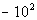(answer/discussion to 3c)Practice Problems 4a - 4b: Find the root.

 4a.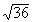(answer/discussion to 4a) 4b.(answer/discussion to 4b)Practice Problems 5a - 5b: Simplify the expression.

 5a.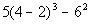(answer/discussion to 5a) 5b.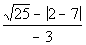(answer/discussion to 5b)Practice Problem 6a: Find the value of the expression when a = 3 and b = -2.

 6a.(answer/discussion to 6a)Need Extra Help on these Topics?

The following are webpages that can assist you in the topics that were covered on this page:

 http://www.purplemath.com/modules/negative.htm This webpage goes over addition, subtraction, multiplication, exponents and roots of real numbers. http://www.purplemath.com/modules/orderops.htm This webpage goes over the order of operations. http://www.mathleague.com/help/posandneg/posandneg.htm#addingpositiveandnegativenumbers This webpage goes over adding, subtracting, multiplying, and dividing signed numbers.  http://www.math.com/school/subject1/lessons/S1U1L11DP.html This webpage helps with adding and subtracting integers. http://www.mathleague.com/help/integers/integers.htm#addingintegers This webpage helps with adding integers. http://www.mathleague.com/help/integers/integers.htm#subtractingintegers This webpage helps with subtracting integers. http://www.math.com/school/subject1/lessons/S1U1L12DP.html This webpage helps with multiplying and dividing integers. http://www.mathleague.com/help/integers/integers.htm#multiplyingintegers This webpage helps with multiplying integers. http://www.mathleague.com/help/integers/integers.htm#dividingintegers This webpage helps with dividing integers. http://home.earthlink.net/~djbach/basic.html#anchor938594 This webpage helps with exponents. http://www.mathleague.com/help/decwholeexp/decwholeexp.htm#exponents This webpage helps with exponents. http://www.blarg.net/~math/squares.html This webpage helps with exponents and square roots. http://home.earthlink.net/~djbach/basic.html#anchor941779 This webpage helps with order of operations.

Go to Get Help Outside the Classroom found in Tutorial 1: How to Succeed in a Math Class for some more suggestions.Last revised on June 10, 2011 by Kim Seward.